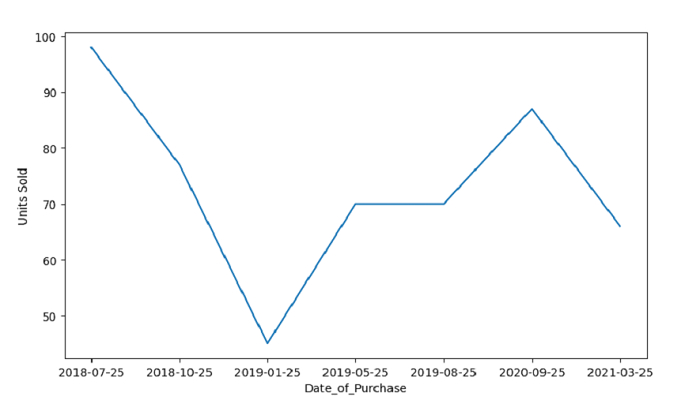# Python - Create a Time Series Plot using Line Plot with Seaborn

To create a Time Series Plot, use the lineplot(). At first, import the required libraries −

import seaborn as sb
import pandas as pd
import matplotlib.pyplot as plt

Create a DataFrame with one of the columns as date i.e. “Date_of_Purchase” −

dataFrame = pd.DataFrame({'Date_of_Purchase': ['2018-07-25', '2018-10-25', '2019-01-25', '2019-05-25', '2019-08-25','2020-09-25','2021-03-25'],'Units Sold': [98, 77, 45, 70, 70, 87, 66]
})

Pot Time Series using lineplot() −

sb.lineplot(x="Date_of_Purchase", y="Units Sold", data=dataFrame)


## Example

Following is the code −

import seaborn as sb
import pandas as pd
import matplotlib.pyplot as plt

# creating DataFrame
dataFrame = pd.DataFrame({'Date_of_Purchase': ['2018-07-25', '2018-10-25', '2019-01-25', '2019-05-25', '2019-08-25','2020-09-25','2021-03-25'],'Units Sold': [98, 77, 45, 70, 70, 87, 66]
})

# time series plot
sb.lineplot(x="Date_of_Purchase", y="Units Sold", data=dataFrame)
plt.show()

## Output

This will produce the following output −